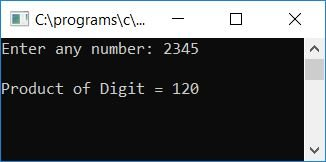# C Program to Find and Print the Product of All Digits of Any Number

Here we will learn about how to create a program in C that will ask the user to enter any number (at run-time) as input and then calculate and print the product of all the digits of that given number. This program will only calculate the non-zero digit's product:

```#include<stdio.h>
#include<conio.h>
int main()
{
int num, prod=1, rem;
printf("Enter any number: ");
scanf("%d", &num);
while(num>0)
{
rem = num%10;
if(rem!=0)
prod = prod*rem;
num = num/10;
}
printf("\nProduct of Digit = %d", prod);
getch();
return 0;
}```

As the program was written in the Code::Blocks IDE, here is the output you will see on your screen after a successful build and run. Let's suppose that the user has supplied the input number as 2345 and pressed the ENTER key:Let's take another sample run:

As you can see from the above sample run, 0 is skipped while multiplying the digits of the given number, as if we take 0 along with a non-zero digit, then the product will be 0.

Here are some of the main steps used in the above program:

• Receive any number as input.
• Create a while loop that runs until the number is greater than 0.
• Inside the for loop, divide the number by 10 to find the remainder, or last digit, of the given number.
• If the current remainder value is not equal to 0, multiply it by the prod variable and set it to the prod variable, which is the product of all the non-zero digits of a number.
• Never forget to give the variable prod the value 1 at the beginning of the program, in the main() function.
• After multiplying the remainder with prod, divide the number by 10 and continue to check and do the same operation.
• Let's suppose that the user has provided the number 247.
• At the first run of the while loop, num holds 247, which is greater than 0.
• The program flow then enters the while loop, where num%10 or 247%10 or 7 is initialized to the rem variable, which is not equal to 0. The program flow then enters the if block (present inside the while loop), where prod*rem or 1*7 or 7 is initialized to prod (prod initially holds 1), and num/10 or 247%10 or 24 is initialized to num.
• Again at the second run of the while loop, that is, when num holds the value 24, which is again greater than 0, then the program flow again goes inside the  while loop and does the same operation as mentioned in the above steps.
• This process continues until the condition of the "while" loop evaluates to false.

C Quiz

« Previous Program Next Program »